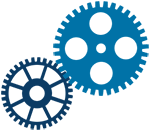ASME PROCESS PIPING - Process Piping: The Complete Guide to ASME B31.3
January 1, 2009 - ASME

ASME B31.3 is the generally accepted standard for process piping, worldwide. It is the de facto standard for piping for the oil, petro-chemical and chemical industries, worldwide. To facilitate its international use, ISO/ WD 15649, Petroleum and natural gas industries-piping, has been...

SEMI PROCESS CHEMICALS - PROCESS CHEMICALS
SEMI
A description is not available for this item.
IEEE MATHEMATICAL METHODS - MATHEMATICAL METHODS IN ARTIFICIAL INTELLIGENCE
IEEE
A description is not available for this item.
MPIF PROCESSING HANDLING - PROCESSING AND HANDLING OF POWDERS AND DUSTS
MPIF
A description is not available for this item.
Signal Processing: A Mathematical Approach
November 12, 2014 - CRC

Signal Processing: A Mathematical Approach is designed to show how many of the mathematical tools the reader knows can be used to understand and employ signal processing techniques in an applied environment. Assuming an advanced undergraduate- or graduate-level...

Sequential Models of Mathematical Physics
February 11, 2019 - CRC

The equations of mathematical physics are the mathematical models of the large class of phenomenon of physics, chemistry, biology, economics, etc. In Sequential Models of Mathematical Physics, the author considers the justification of the process of constructing...

Physico-Mathematical Theory of High Irreversible Strains in Metals
February 1, 2019 - CRC

Presents a new physical and mathematical theory of irreversible deformations and ductile fracture of metals that acknowledges the continuous change in the structure of materials during deformation and the accumulation of deformation damage. Plastic deformation, viscous destruction, evolution...

Mathematical Principles of the Internet, Volume 1: Engineering
December 13, 2018 - CRC

This two-volume set on Mathematical Principles of the Internet provides a comprehensive overview of the mathematical principles of Internet engineering. The books do not aim to provide all of the mathematical foundations upon which the Internet is based. Instead, they cover a...

A Transition to Proof: An Introduction to Advanced Mathematics
April 1, 2019 - CRC

A Transition to Proof: An Introduction to Advanced Mathematics describes writing proofs as a creative process. There is a lot that goes into creating a mathematical proof before writing it. Ample discussion of how to figure out the "nuts and bolts'" of the proof takes place:...

Advanced Construction Mathematics
April 9, 2019 - CRC

Advanced Construction Mathematics covers the range of topics that a student must learn in order to achieve success in Level 3 and 4 mathematics for the Pearson BTEC National and BTEC HNC/HND in Construction, Building Services, and Civil Engineering. Packed with easy to follow...

Theory of Spatial Statistics: A Concise Introduction
March 22, 2019 - CRC

Theory of Spatial Statistics: A Concise Introduction presents the most important models used in spatial statistics, including random fields and point processes, from a rigorous mathematical point of view and shows how to carry out statistical inference. It contains full proofs,...

Modeling and Simulation of Chemical Process Systems
November 6, 2018 - CRC

In this textbook, the author teaches readers how to model and simulate a unit process operation through developing mathematical model equations, solving model equations manually, and comparing results with those simulated through software. It covers both lumped parameter systems and...

Mathematical Statistics and Stochastic Processes
January 1, 2012 - WILEY

This book is devoted to Mathematical Statistics and Statistics for Stochastic Processes, based on Measure theory, Probability theory and Decision theory. Contents: Decision theory, Estimation, Tests, Confidence intervals, asymptotic Statistics, nonparametric Statistics and robustness,...

Manufacturing: Mathematical Models, Problems, and Solutions
May 16, 2018 - CRC

This book is written for readers who are either practicing engineers in industry or engineering-degree students taking a course in manufacturing technology. The book is divided into three parts which includes problems and solutions in basic manufacturing processes, problems and solutions in...

ASTM D3370 - Standard Practices for Sampling Water from Flowing Process Streams
December 15, 2018 - ASTM

These practices cover the equipment and methods for sampling water from closed conduits such as process streams at power stations for chemical, physical, microbiological, and radiological analyses. It does not cover specialized equipment required for and unique to a specific test or method...

The Beauty of Mathematics in Computer Science
November 5, 2018 - CRC

The Beauty of Mathematics in Computer Science explains the mathematical fundamentals of information technology products and services we use every day, from Google Web Search to GPS Navigation, and from speech recognition to CDMA mobile services. The book was published in Chinese in...

Chemical Process Design and Simulation: Aspen Plus and Aspen Hysys Applications
December 1, 2018 - WILEY

A comprehensive and example oriented text for the study of chemical process design and simulation Chemical Process Design and Simulation is an accessible guide that offers information on the most important principles of chemical engineering design and includes illustrative examples of...

Advertisement